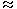1.  The strength of school increased/decreased from 1997 to 1998 by approximately what percent?

 A. 1.2% B. 1.7% C. 2.1% D. 2.4% E. 2.6%

Explanation:

Before solving the questions, we shall analyse the graph :

From the graph it is clear that :

In 1996 : Number of students left = 250 and number of students joined = 350.

In 1997 : Number of students left = 450 and number of students joined = 300.

In 1998 : Number of students left = 400 and number of students joined = 450.

In 1999 : Number of students left = 350 and number of students joined = 500.

In 2000 : Number of students left = 450 and number of students joined = 400.

In 2001 : Number of students left = 450 and number of students joined = 550.

Therefore, the numbers of students studying in the school (i.e., strength of the school) in various years:

In 1995 = 3000 (given).

In 1996 = 3000 - 250 + 350 = 3100.

In 1997 = 3100 - 450 + 300 = 2950.

In 1998 = 2950 - 400 + 450 = 3000.

In 1999 = 3000 - 350 + 500 = 3150.

In 2000 = 3150 - 450 + 400 = 3100.

In 2001 = 3100 - 450 + 550 = 3200.

Percentage increase in the strength of the school from 1997 to 1998

 =(3000 - 2950) x 100% = 1.69%1.7%. 2950